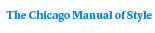# Fuchsian Groups

Svetlana Katok186 pages | 30 line drawings, 1 table | 5-1/4 x 8 | © 1992
Paper \$32.00 ISBN: 9780226425832 Published August 1992
Cloth \$77.00 ISBN: 9780226425825 Published August 1992
This introductory text provides a thoroughly modern treatment of Fuchsian groups that addresses both the classical material and recent developments in the field. A basic example of lattices in semisimple groups, Fuchsian groups have extensive connections to the theory of a single complex variable, number theory, algebraic and differential geometry, topology, Lie theory, representation theory, and group theory.
Table of Contents• Contents
Contents
Preface
1. Hyperbolic geometry
1.1. The hyperbolic metric
1.2. Geodesics
1.3. Isometrics
1.4. Hyperbolic area and the Gauss-Bonnet formula
1.5. Hyperbolic trigonometry
1.6. Comparison between hyperbolic, spherical and Euclidean trigonometry
Exercises for Chapter 1
2. Fuchsian groups
2.1. The group PSL(2,R)
2.2. Discrete and properly discontinuous groups
2.3. Algebraic properties of Fuchsian groups
2.4. Elementary groups
Exercises for Chapter 2
3. Fundamental regions
3.1. Definition of a fundamental region
3.2. The Dirichlet region
3.3. Isometric circles and the Ford fundamental region
3.4. The limit set of [ ]
3.5. Structure of a Dirichlet region
3.6. Connection with Riemann surfaces and homogeneous spaces
Exercises for Chapter 3
4. Geometry of Fuchsian groups
4.1. Geometrically finite Fuchsian groups
4.2. Cocompact Fuchsian groups
4.3. The signature of a Fuchsian group
4.4. Fuchsian groups generated by reflections
4.5. Fuchsian groups of the first kind
4.6. Finitely generated Fuchsian groups
Exercises for Chapter 4
5. Arithmetic Fuchsian groups
5.1. Definitions of arithmetic Fuchsian groups
5.2. Fuchsian groups derived from quaternion algebras
5.3. Criteria for arithmeticity
5.4. Compactness of [ ] for Fuchsian groups derived from division quaternion algebras
5.5. The modular group and its subgroups
5.6. Examples
Exercises for Chapter 5
Hints for Selected Exercises
Bibliography
Index